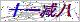••Product classification

Related article

# 西门子6RA70三相不平衡故障维修中心

## 6RA70当天修好西门子6RA70三相不平衡故障维修中心的详细资料：

`内外控参数的设置 P055=112，P057=112，将1#组参数拷贝到2#组，选择内外控观察R056， R058参数组选择情况 P171.2= 150%，P172.2= -150% P644.2=3002 外控速度给定。 P648.01(B)= 9P648.02(B)= 3001 内外控时控制字的选择 P649.01(B)=9 P649.02(B)= 9 P676.1(B)=P676.2=17用开关量输入端子39作为功能数据组FDS选择P677.1(B)=P677.2=0.（选择3#组、4#组，该选择功能禁止） P690.1(B)=P690.2=17用开关量输入端子39作为功能数据组BDS选择 P641.1(B)=P641.2=17用开关量输入端子39作为选择斜坡函数发生器旁路与否内控状态选1#组，外控状态选2#组.，或令P641.01=0， P641.02=1    西门子6RA70三相不平衡故障维修中心十四. 通讯参数的设定通讯板CBP是小板附着在ADB板上，PC机需要数据文件SIEM8045.GSD P918=通讯站号地址设定，PLC对应同样站地址 U711.1=0 U712= 2 定义通讯字类型为 4PKW+6PZD U722=0 禁止通讯故障F082 P927=7 CBP+PMU+G-SST1+OP1S控制接口的使能 U710.1=0 激活CBP配置 PLC到传动的信号`

### 留言框

• #### 验证码：请输入计算结果（填写阿拉伯数字），如：三加四=7